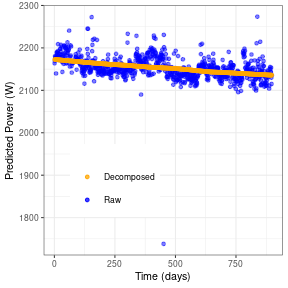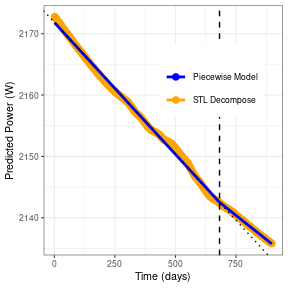# Non-linear Performance Loss Rates

Typical PLR values are reported as a single number, such as $$-0.5\%/yr$$, that is meant to represent the trend of the total available time-series. While this is an easily interpretable result and is useful for warrenty trends which guarentee certain power outputs after a given amount of use, it is based on the assumption that the system is changing in a linear fashion. This assumption can lead to biases in results, especially considering that there are several well known causes of power loss that are not linear, including seasonal soiling or light indiced degradation. In particular, light induced degradation has led to PERC modules typically having two rated power loss warrenties, one for the first year and one for after the first year. The first year is expected to have much higher power loss while the module saturates and stabilizes, with less power loss after a period of exposure. This information can be lost in a linear assumption of PLR, so it is beneficial to include functionality to automatically evaluate the non-linear trends in PLR for a given system, especially in a PV research setting.

This function has been desgined to provide an easy to use and interpret method for evaluation non-linear trends in PLR calculations that can be integrated into assumed linear PLR calculation pipelines.

To maintain a high degree of interpretability, non-linear PLR is evaluated using piecewise linear modeling, through the segmented package available in R. Piecewise linear modeling divides a time-series into a certain number of different linear models at a series of change points. All the models start and end at change points, with the exception of the Y-intercept and the last data point. Each individual PLR value can is the same as a linear PLR value, but instead of a single linear value a time-series is representated by a series of different linear PLR values, creating a non-linear trend. This is similar to the more recent ratings for PERC modules with different PLR values for the first year and the rest of the modules lifetime.

The change points are detected automatically based on model best fits. The user specifies how many change points the final model will have.

An important note is that time-series with seasonality will often not give good piecewise linear fits as the change points will fit to the seasonal trend, not the overall time-series. As such, it is highly recommended to decompose any time-series before running a non-linear PLR evaluation. Keep in mind the decomposition will tend to smooth out sharp jumps in the time-series, which may bias results depending on the nature of individual systems.

An example of a typical non-linear PLR evaluation is given below.

First build a weather corrected predicted power time-series as you would for any PLR analysis; any model choice will work. We will then decompose this time-series to prevent seasonality influence.


predict_data <- data.frame(irrad_var = 800, temp_var = 40)

weather_cor_utc <- PVplr::plr_xbx_utc_model(test_dfc, var_list, by = "day", data_cutoff = 10, ref_irrad = 800, irrad_range  = 20, predict_data = predict_data)

decomp <- PVplr::plr_decomposition(data = weather_cor_utc, freq = 365, power_var = "power_var", time_var = "time_var")

ggplot() +
geom_point(data = decomp, aes(x = age, y = raw, color = "Raw"), alpha = 0.5, size = 1) +
geom_point(data = decomp, aes(x = age, y = trend, color = "Decomposed"), size = 1, alpha = 0.5) +
scale_color_manual(values = c("orange", "blue")) +
labs(x = "Time (days)", y = "Predicted Power (W)", color = "") +
theme_bw(base_size = 8) +
theme(legend.position = c(0.3, 0.3))Comparing the trends we see some yearly seasonality as well as a few outliers that are removed during the decomposition. Initial observation seems to show that the trend for this system is fairly linear, with no major shifts in performance.

A quick runthrough of linear PLR gives $$-0.69\%/yr$$ for regression and $$-0.68\%/yr$$ for YoY, very similar values.

# multiple assumed linear methods of calculating PLR
PVplr::plr_weighted_regression(decomp, power_var = "trend", time_var = "age", per_year = 365, weight_var = "weights", model = "utc")
#>            plr       error model   method
#> tvar -0.693921 0.001397359   utc weighted
PVplr::plr_yoy_regression(decomp, power_var = "trend", time_var = "age", per_year = 365, model = "utc")
#>          plr     plr_sd model       method
#> 1 -0.6844849 0.03505701   utc year-on-year

The plr_seg_extract() function requires the predicted power time-series, the number of data points per year (as in YoY), the desired number of breakpoints, the names of the power and time variables, and lastly whether the segmented model of PLR result should be returned. return_model defaults to FALSE which returns the PLR results for each segment. Returning the model itself is useful for additional analysis or making piecewise plots.

# evaluate segmented PLR results
seg_plr_result <- PVplr::plr_seg_extract(df = decomp, per_year = 365, n_breakpoints = 1, power_var = "trend", time_var = "age")

seg_plr_result
#>          plr seg_start  seg_end       plr_sd segment     yint   Adj_R2
#> 1 -0.7223111    0.0000 682.0675 0.0016532648       1 2171.825 0.998303
#> 2 -0.5272254  682.0675 898.0000 0.0009903878       2 2171.825 0.998303

# return segmented model instead of PLR result
model <- PVplr::plr_seg_extract(df = decomp, per_year = 365, n_breakpoints = 1, power_var = "trend", time_var = "age", return_model = TRUE)

# predict data along time-series with piecewise model for plotting
pred <- data.frame(age = seq(1, max(decomp$age, na.rm = TRUE), length.out = 10000)) pred$seg <- predict(model, newdata = pred)

# plot segmented model along decomposed time series
# add initial PLR trend as well
ggplot() +
geom_point(data = decomp, aes(x = age, y = trend, color = "STL Decompose"), size = 2) +
geom_line(data = pred, aes(x = age, y = seg, color = "Piecewise Model"), size = 1) +
geom_abline(slope = seg_plr_result$plr/(100*365)*seg_plr_result$yint, intercept = seg_plr_result$yint, linetype = "dotted") + scale_color_manual(values = c("blue", "orange")) + geom_vline(xintercept = seg_plr_result$seg_end, linetype = "dashed") +
labs(x = "Time (days)", y = "Predicted Power (W)", color = "") +
theme_bw(base_size = 8) +
theme(legend.position = c(0.7, 0.7))
#> Warning: Using size aesthetic for lines was deprecated in ggplot2 3.4.0.
#> ℹ Please use linewidth instead.Non-linear PLR results show PLR values of $$-0.87 \%/yr$$ for the first 182 time segments (days in this case), followed by a smaller PLR of $$-0.67\%/yr$$ after that period, indicating evidence of higher losses during the start of this systems performance.

Individual time segments and uncertainties are given for each PLR result. This segmented model gives a very strong with with the decomposed trend of the time-series, with an $$Adj-R^2$$ over $$0.99$$. As of this time we do not yet have a bootstrap uncertainty application for piecewise PLE calculations, we are currently working on the best ways to represent PLR uncertainty for all different calculation methods with future updates planned to expand on this.

## Sources

V. M. R. Muggeo, “Segmented: Regression Models with Break-Points / Change-Points Estimation,” Nov. 2017.

V. M. Muggeo, “Segmented: An R package to fit regression models with broken-line relationships,” R news, vol. 8, no. 1, pp. 20–25, 2008.